Test: Synchronous Machine of Electrical Machines

# Test: Synchronous Machine of Electrical Machines

Test Description

## 10 Questions MCQ Test GATE Electrical Engineering (EE) 2023 Mock Test Series | Test: Synchronous Machine of Electrical Machines

Test: Synchronous Machine of Electrical Machines for Electrical Engineering (EE) 2023 is part of GATE Electrical Engineering (EE) 2023 Mock Test Series preparation. The Test: Synchronous Machine of Electrical Machines questions and answers have been prepared according to the Electrical Engineering (EE) exam syllabus.The Test: Synchronous Machine of Electrical Machines MCQs are made for Electrical Engineering (EE) 2023 Exam. Find important definitions, questions, notes, meanings, examples, exercises, MCQs and online tests for Test: Synchronous Machine of Electrical Machines below.
Solutions of Test: Synchronous Machine of Electrical Machines questions in English are available as part of our GATE Electrical Engineering (EE) 2023 Mock Test Series for Electrical Engineering (EE) & Test: Synchronous Machine of Electrical Machines solutions in Hindi for GATE Electrical Engineering (EE) 2023 Mock Test Series course. Download more important topics, notes, lectures and mock test series for Electrical Engineering (EE) Exam by signing up for free. Attempt Test: Synchronous Machine of Electrical Machines | 10 questions in 30 minutes | Mock test for Electrical Engineering (EE) preparation | Free important questions MCQ to study GATE Electrical Engineering (EE) 2023 Mock Test Series for Electrical Engineering (EE) Exam | Download free PDF with solutions
 1 Crore+ students have signed up on EduRev. Have you?
Test: Synchronous Machine of Electrical Machines - Question 1

### Match List-I (Name of test) with List-II (Result) and select the correct code given below the lists:  List-I A. Open Circuit and short circuit test B. Open circuit and zero power factor test C. Slip test D. Maximum lagging current test List-II 1. Leakage reactance 2. Direct axis synchronous reactance 3. Quadrature axis synchronous reactance 4. Ratio of direct axis synchronous reactance to quadrature axis synchronous reactance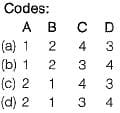Test: Synchronous Machine of Electrical Machines - Question 2

### The phasor diagram of a synchronous machine connected to an infinite bus is shown below. The machine is acting as a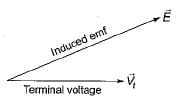Detailed Solution for Test: Synchronous Machine of Electrical Machines - Question 2

As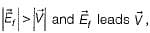therefore machine is acting as a generator and operating at lagging Pf.

Test: Synchronous Machine of Electrical Machines - Question 3

### A 6-pole alternator with 36 slots carries a 3-phase distributed winding. Each coil is short-pitched by one slot. The winding factor is given by

Detailed Solution for Test: Synchronous Machine of Electrical Machines - Question 3

Number of slots/poIe/phase,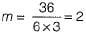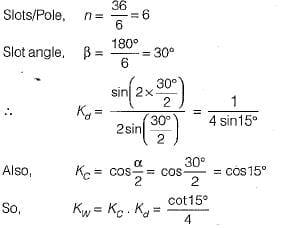Test: Synchronous Machine of Electrical Machines - Question 4

A winding is distributed in the slots along the air-gap periphery
1. to add mechanical strength to the winding.
2. to reduce the amount of conductor material required.
3. to reduce the harmonics in the generated emf.
4. to reduce the size of the machine.
5. for full utilization of iron and conductor materials.
From these, the correct answer is

Test: Synchronous Machine of Electrical Machines - Question 5

Which of the following graphs represents the speed-torque characteristic of a synchronous motor?

Detailed Solution for Test: Synchronous Machine of Electrical Machines - Question 5

For a synchronous motor, N = Ns for all value of torque.

Test: Synchronous Machine of Electrical Machines - Question 6

In figure the characteristic that corresponds to the variation of synchronous reactances of synchronous machine with the field current is​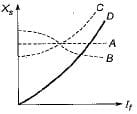Test: Synchronous Machine of Electrical Machines - Question 7

The four methods of calculating voltage regulation of a 3-phase alternator are:
1. Emf method
2. Saturated synchronous reactance method
3. New ASA method
4. Mmf method
The correct sequence in the ascending order of the values of regulation obtained by these methods is

Test: Synchronous Machine of Electrical Machines - Question 8

The flux per pole in a synchronous motor with the field circuit ON and the stator disconnected from the supply is found to be 25 mWb. When the stator is connected to the rated supply with the field excitation unchanged, the flux per pole in the machine is found to be 20 mWb while the motor is running on no-load. Assuming no-load losses to be zero, the no-load current drawn by the motor from the supply

Detailed Solution for Test: Synchronous Machine of Electrical Machines - Question 8

Since after connecting the stator to supply, flux per pole is reduced to 20 mWb from initial value of 25 mWb, therefore effect of armature reaction is demagnetizing i.e. Ff > Fr Due to which motor is over-excited and runs at leading power factor.

Test: Synchronous Machine of Electrical Machines - Question 9

Which of the following method would give a lower than actual value of regulation of an alternator?

Detailed Solution for Test: Synchronous Machine of Electrical Machines - Question 9

Mmf method is called optimistic method because it gives voltage regulation lower than the actual value.

Test: Synchronous Machine of Electrical Machines - Question 10

A synchronous motor is operating on no-load at unity power factor. If the field current is increased, the pf will become

Detailed Solution for Test: Synchronous Machine of Electrical Machines - Question 10

Motor becomes over-excited and starts operating at leading pf with the increase in field current.
Here, Ia will increase.

## GATE Electrical Engineering (EE) 2023 Mock Test Series

22 docs|274 tests
 Use Code STAYHOME200 and get INR 200 additional OFF Use Coupon Code
Information about Test: Synchronous Machine of Electrical Machines Page
In this test you can find the Exam questions for Test: Synchronous Machine of Electrical Machines solved & explained in the simplest way possible. Besides giving Questions and answers for Test: Synchronous Machine of Electrical Machines, EduRev gives you an ample number of Online tests for practice

## GATE Electrical Engineering (EE) 2023 Mock Test Series

22 docs|274 tests